Courses
Courses for Kids
Free study material
Free LIVE classes
More

# Area Model MultiplicationLIVE
Join Vedantu’s FREE Mastercalss

## Introduction to Area Model

Multiplication is a fundamental arithmetic operation that students learn at a young age. If they have a keen understanding of multiplication, they can start applying this concept to real-world problems. The area model multiplication worksheets consist of various questions that help students to visualise multiplication. With the help of the given solved examples, students will understand the area model of multiplication more clearly. Some practice problems are given that need to be solved by the students on their own, to develop a better understanding of the topic.

## What is the Multiplication with Area Model?

In mathematics, an area model is defined as a rectangular diagram or model which is used in multiplication and division problems, where the factors or the quotient and divisor specify the length and width of the rectangle. One can break one large area of the rectangle into several smaller boxes, using number bonds, to make the calculation easier. Then add the number to get the area of the whole, which is the product.

By using the area model we can multiply 2 two-digits numbers by following the given steps: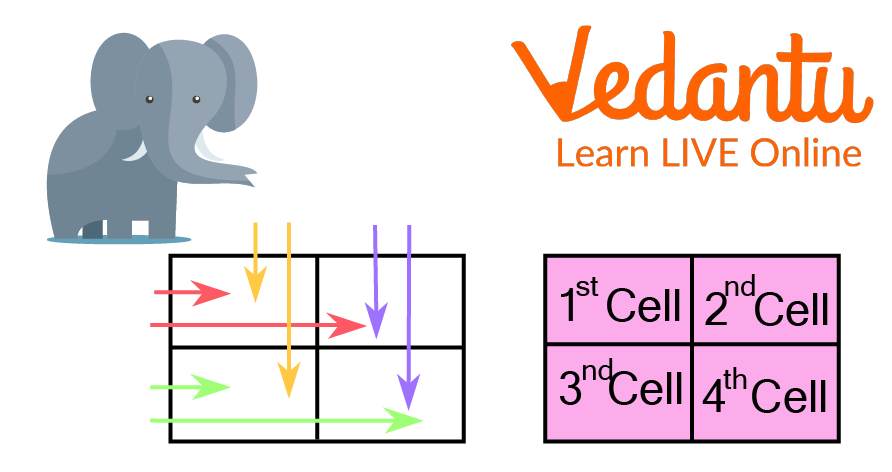Multiplication with Area Model

Here, as an example, the area model has been used to multiply 27 and 35.

• Write the multiplicands in expanded form as tens and ones. For example, 27 is 20 and 7, and 35 is 30 and 5.

• Draw a 2 × 2 grid, which is a box with 2 rows and 2 columns.

• Write the terms of 1 of the multiplicands on the highest of the grid (box). One of the highest of every cell.

• On the left of the grid write the terms of the other multiplicand. One on the side of every cell.

• Write the product of the number on the tens in the first cell. Then write the product of the tens and ones in the second and third cells. Write the product of the ones in the fourth cell.

• Lastly, to get the final product, add all the partial products.

Thus, The multiplication of 27 and 35 is 945.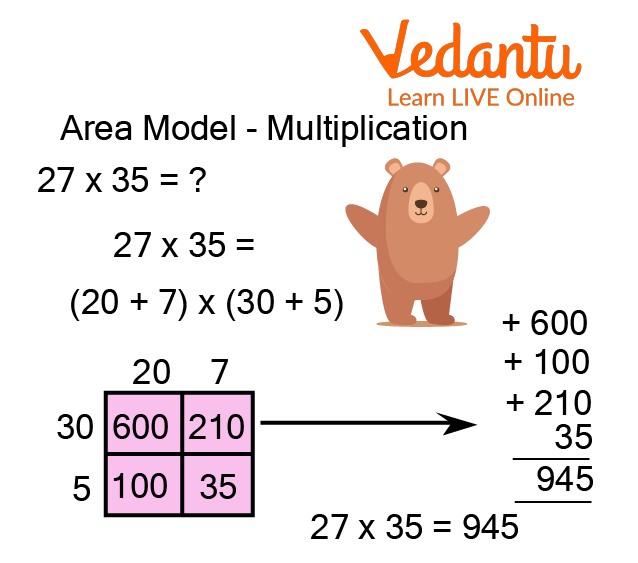Area Model Multiplication

## Multiplying with Area Model a 1-Digit Number

One can use area models in multiplying large numbers. Display the problem as the area of a rectangle, and then break that rectangle into smaller parts to solve it easily. This method is also known as the box multiplication method. Now, try it with 628 × 5. Let the problem be shown as the area of a rectangle:

Next, let the rectangle split into smaller pieces for easier multiplication. We will use the expanded form of 628 to keep the maths simple:

628 = 600 + 20 + 8

Now, multiply to find the area of each smaller rectangle. Lastly, to find the area of the original rectangle adds those products.

3000 + 100 + 40 = 3140

So, the product 628 × 5 is equal to 3140.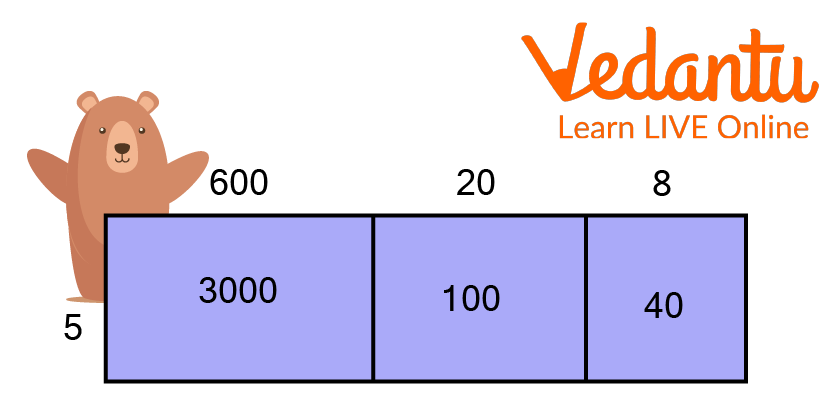Multiplying with The Area Model

## Multiplying with Area Model a 2-Digit Number

One can use area models for larger problems, too. Let's use 36×52 as an example. Firstly, write each factor in expanded form, i.e.

36 = 30 + 6

52 = 50 + 2

To find the area of each smaller rectangle, multiply the above expansion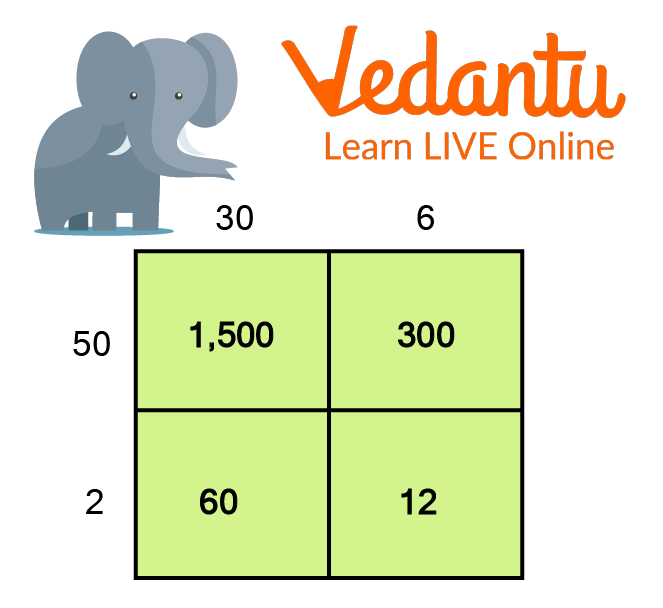On Multiplying The Above Expansion

Lastly, add those products to find the total area.

1500 + 300 + 60 + 12 = 1872

So, the required product of 36 × 52 is 1872.

## Solved Examples

Q1. Multiply Using Area Model

(1). 6 x 24

Ans: Multiplication with area model of the term, 6 x 24 is given by: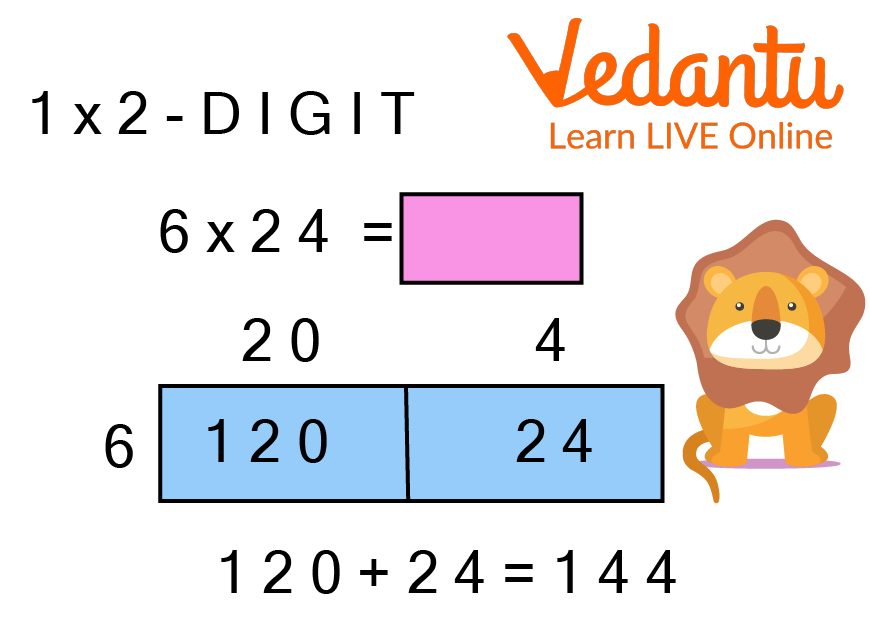Multiplication of 6 and 24

Thus, the multiplication of 6 x 24 using the area model is 144.

(2). 4 x 125

Ans: Multiplication with area model of the term, 4 x 125 is given by: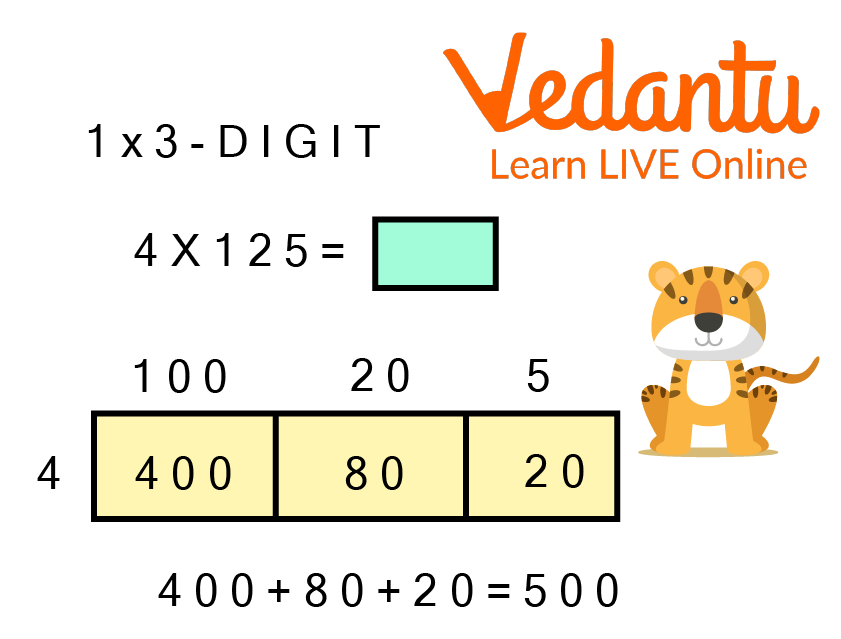Multiplication of 4 and 125

Thus, the multiplication of 4 x 125 using the area model is 500.

Q1. 94 x 12

Ans: 1128.

Q2. 70 x 13

Ans: 910.

Q3. 69 x 36

Ans: 2484.

## Summary

In this article, we have learned about the Area Model Multiplication. In area model multiplication, a rectangular diagram or model is used for solving multiplication and division problems. In this article, we have learned to multiply a 1-digit number & a 2-digit number using an area model. Lastly, we have also learned about some fun facts related to multiplying with the area model. Some solved examples based on the area model are being discussed for the clarity of concepts. Practice problems are also assigned, along with their answers.

Last updated date: 01st Oct 2023
Total views: 95.7k
Views today: 0.95k

## FAQs on Area Model Multiplication

1. What is the area of a circle?

Area of a circle = $\pi r^2$, where r is the radius of a circle.

2. What is the area of the triangle?

Area of the triangle = $\dfrac{1}{2} \times b \times h$, where b is the base of the triangle and h is the height of the triangle.

3. What is the unit of area?

The area is measured in terms of the square of units, such as square feet, square centimetres, square metres, etc.